Mathematics Questions for NDA, CDS & CAPF: 13th August

Dear Students, Defence Adda is providing you all with this quiz on Mathematics questions for NDA, CDS, AFCAT, CAPF and other Police Examinations. The questions asked in Mathematics Section of most of the defence examinations are based on the topics from Mathematics of Class 11th and 12th. The questions asked in this section are complex and comparatively difficult but once attempted with high accuracy, can fetch you full marks in this section. Also, practice on a daily basis helps one dive into the core concepts of a subject and thus, help her perform to the best of her ability in the real examinations. So, attempt the daily quizzes being provided by Defence Adda and score to the maximum in Mathematics Section of all sorts of defence examinations.

Q1. If θ is the acute angle between the diagonals of a cube, then which one of the following is correct?
(a)  θ = 30°
(b) θ = 45°
(c) 2cos θ = 1
(d) 3cos θ = 1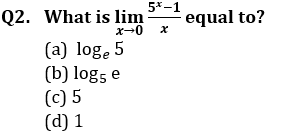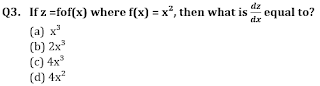Q5. The average age of 20 students in a class is 15 yr. If the teacher’s age is included, the average increase by one. What is the teacher’s age?
(a)  30 yr
(b) 21 yr
(c)  42 yr
(d) 36 yr

Q6. The line 2y=3x+12 cuts the parabola 4y=3x² .what  is the area enclosed by the parabola and the line?
(a) 27 sq units
(b) 36 sq units
(c) 48 sq units
(d) 54 sq units

Q7. Locus of the centre of the circle which always passes through the fixed points (a, 0) and (–a, 0) is
(a)  x= 1
(b) x + y = 6
(c) x + y = 2a
(d) x = 0

Q9. Consider the following statements
I. Every zero matrix is a square matrix.
II. A matrix has a numerical value.
III. A unit  matrix is a diagonal matrix.
Which of the above statement(s) is/ are correct?
(a)  Only II
(b) Only III
(c) Both II and III
(d) Both I and III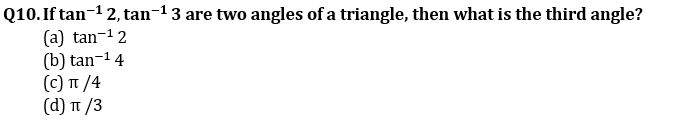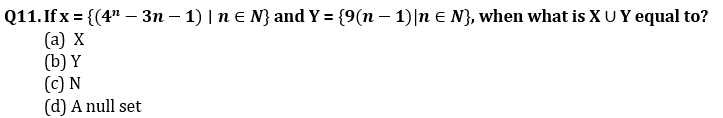Q13. From an aeroplane above a straight road the angles of depression of two positions at a distance 20 m apart on the road are observed to be 30° and 45°. The height of the aeroplane above the ground is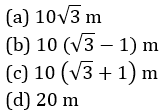Solutions